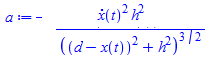## Error: " cannot determine if this expression is tr...

I'm trying to execute the linked code but i am having the following error:

Error, (in sombrea2) cannot determine if this expression is true or false: -(1/4)*105^(1/2) <= (1/4)*105^(1/2)

If someone can help me to find a solution I'll be very thankful.

## how do you assume a positive solution for a system...

I was working on a project about optimal strategies for HIV treatment, models used from [Butler, Kirschner, and Lenhart] 1997. This model explains the spread of HIV viruses in the human body, where there is one control function u(t).My work is following pontryagin maximum principle. But i have a problem solving the differential equation system, where there are 6 differential equations with 6 initial conditions. Everything works normally and I get a numerical solution for the system

```restart;
with(linalg);
with(DEtools);
with(plots);
```

Model declaration

```dx := -T*V*k*u+B*T*r-T*m1+A; dx := T*V*k*u-Ti*m2; dx := N*Ti*m2-V*m3; H := A*T-(1-u)^2+add(dx[i]*L[i], i = 1 .. 3); satu := -(diff(H, T)); dua := -(diff(H, Ti)); tiga := -(diff(H, V)); empat := diff(H, L); lima := diff(H, L); enam := diff(H, L)

eq1 := diff(L1(t), t) = -A-(-V(t)*k*u(t)+B*r-m1)*L1(t)-u(t)*k*V(t)*L2(t); eq2 := diff(L2(t), t) = -N*m2*L3(t)+m2*L2(t); eq3 := diff(L3(t), t) = T(t)*k*u(t)*L1(t)-T(t)*k*u(t)*L2(t)+m3*L3(t); eq4 := diff(T(t), t) = -T(t)*V(t)*k*u(t)+B*T(t)*r-T(t)*m1+A; eq5 := diff(Ti(t), t) = T(t)*V(t)*k*u(t)-Ti(t)*m2; eq6 := diff(V(t), t) = N*Ti(t)*m2-V(t)*m3

```

Value for parameter

``` u(t):=-1/(2)*L1(t)*k*V(t)*T(t)+1/(2)*L2(t)*k*V(t)*T(t)+1; s:=10;  m1:=0.02;  m2:=0.5;  m3:=4.4;  r:=0.03;  Tm:=1500;  k:=0.000024;  N:=300;    A:=1;  B:=1-(T(t)+Ti(t))/(Tm):
```

Numerical Solution with BVP

`fcns := {L1(t), L2(t), L3(t), T(t), Ti(t), V(t)}; a := dsolve({eq1, eq2, eq3, eq4, eq5, eq6, L1(20) = 0, L2(20) = 0, L3(20) = 0, T(0) = 800, Ti(0) = 0.4e-1, V(0) = 1.5}, fcns, type = numeric, method = bvp[midrich])`

The plot

`odeplot(a, [[t, u(t)], [t, V(t)]], 0 .. 20, numpoints = 1000)`

OutputBlue: V(t)
Red: u(t)
Based on graphs v (t) and u (t) have negative values, whereas in fact v (t) shows many viruses where it is never negative (this is irrational) and u (t) is limited in interval [0,1]. My question are:
How to provide positive assumptions for the system solution?
So v (t), T (t), Ti (t) are never negative?

## guess vector in fsolve...

Hello.

Can you please tell how the guess vector is defined in Newton's method in "fsolve" if not set initial interval for unknowns? Maybe someone knows what "norm of errors", "new norm" and "incr" in "infolevel[fsolve]" are?

Thanks!

## power of integers...

In my expresions I have an integer, nx, which actually has values of only +1 and -1 but I do not specify which.

THe results come out as powers of nx, say nx^n, where n is a positive integer.

How do I reduce the expression nx^n,= 1 for n even and nx^n,= nx for n odd?

## Plotting bifurcation diagram for linear system wit...

I have the following system

```pendsys := { diff(x(t),t) = a * x(t) + y(t), diff(y(t),t) = -x(t) + a * y(t) }:
```

with critical point being (0,0). After plotting the phase portrait, I found out that (0,0) is (asymptotically) stable when a<0 and unstable when a>0. Also, (0,0) is a center when a = 0. Also, as initial condition, I have x(0)=0, y(0)=1. I was thinking we have Hopf bifurcation at a = 0. My question is, how do I plot the bifurcation diagram for this system?

tes_A.mwtes_A.mw

## pdsolve gives wrong extra arguments: {method = Fou...

I undertsand method=Fourier needs boundary conditions to work, but I do not think this error message is right. Compare

```restart;
pde := diff(u(r, theta), r, r)+diff(u(r, theta), theta, theta) = 0;
iv := u(2, theta) = 3*sin(2*theta)+1;
pdsolve([pde,iv], u(r,theta), method = Fourier)```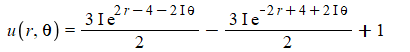With

```restart;
pde := diff(u(r, theta), r, r)+diff(u(r, theta), theta, theta) = 0;
pdsolve(pde, u(r,theta), method = Fourier)```

Error, (in pdsolve/info) wrong extra arguments: {method = Fourier}

pdsolve should return no solution instead. The way it is above, I thought at first I had wrong syntax with the "method = Fourier" settings and I think this error message can be misleading to a user.

## VectorCalculus, PlotVector...

Why doesn't PlotVector plot the arrows all in one size?  (as seen in the Maple help page here)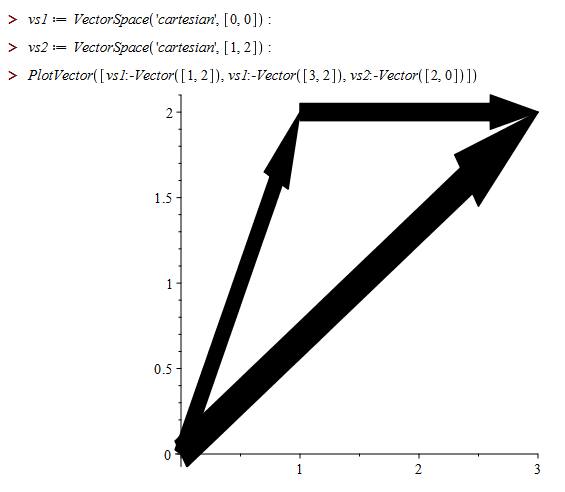## odeplots in an Explore command...

Dear Maple users

Sometimes I use the Explore command in order to watch how plots containing parameters change when the parameters are changed via sliders. I tried to do the same with an odeplot. I can make it work, but I cannot do it the same way as I use to do with ordinary plots: I like to define the plot outside the Explore command, because those Explore commands can be quite cluttered and hard to overview. It is not that bad in my attached example below. Sometimes I have display commands handling several plots ...

In the attached file I show first how it works when the odeplot is inside the Explore command, but when I later move it outside, it won't work. Why this difference between ordinary plots and odeplots? Or maybe there is a workaround to make it work having the odeplot command outside ...

Regards,

Erik

odeplots_in_an_Explore_command.mw

## Solving equations based on numeric solution of ODE...

Dear Maple users

Some ODE's like the Lotka Volterra system can only be solved numerically. I would like to solve some equations based on the numerical solution to that system. Let the x(t) and y(t) be the solutions to the ODE system.

Now, obviously it won't work afterwards to write an equation like solve(x(t)=60,t), since the solution of the ODE only exists as a procedure. Of course I can make an odeplot and zoom in to get an approximate solution to x(t)=60. I would however like it to done automatically. How is this done in the most convenient way when the soltuion of the ODE is given as a procedure?

Example attached.

Regards

Erik

Lotka_Volterra.mw

## Predict Perfect...

Hi

Initially I tried to find pemutations of 1..9, ie [[1,2,3],[4,5,6],[7,8,9]],[[1,2,4],[3,5,6],[7,8,9]],...etc, But maybe not all of them?

I wonder if someone out there can change my code to reflect the following:

1/According to the pdf excerpt, there are a total of 199 unique sums, the smallest sum is 774 and the largest 2556.(From displaying 280 outcomes, the code got the 774).

2/Show which sums have the greatest probability (specifically, 1566, 1575, 1638, 1656, 1674, 1692, 1755, and 1764, each of which can be shown with effort to have a 3/280, or 1.07%, probability – the calculations to determine this probability is a brute-force computation, and requires enumerating all outcomes of the sample space within a computational software package). We then finally reveal the prediction, which of course is correct..(I don't get it)

predict_perfect.mw

## How to apply a function to two unequal length list...

I want to divide every element of A1 list by every element of list A2. Lists are unequal length.

Example:

A1:= [a1, a2, a3];

A2:= [b1, b2];

Output would be:

[a1/b1, a2/b1, a3/b1, a1/b2, a2/b2, a3/b2]

I managed to do this by this means:

LinearAlgebra:-OuterProductMatrix([a1, a2, a3], [1/b1, 1/b2]); convert(%, list)

My questions are:

1. Is there a more succinct, practical method?
2. How about if I want to use a different operation like say adding or just use a function f?

## what is difference between the following syntax f...

I've been using the following syntax to set boundary condition which is a derivative, when passing it to pdsolve. Say we want to set u(r,theta,t) to have insulated boundary conditions at r=1. So the BC will be

For example, to set derivative of u w.r.t. "r" to zero when r=1

eval(  diff(u(r,theta,t),r), r=1) = 0;  #(1)

or using this syntax

D(u)(1, theta, t) = 0;  #(2)

But now I find, on one example below, that the above no longer works.  I have to use this syntax (which I did not know about) for it to work

D*u(1, theta, t) = 0;  #(3)

Has something changed? why when using (3) pdsolve now gives result, but when using (2) or (1) it returns unevaluated? are the three semantically equivalent? when to use which syntax?

I am using Physics updates 265, Latest Maple 2018.2

Here is an example showing the (1,2)  syntax no longer works, but the (3) syntax works

```#articolo example 6.9.2
restart;

#using (1) syntax
pde := diff(u(r, theta, t), t) = (diff(u(r, theta, t), r)+r*(diff(u(r, theta, t), r, r))+(diff(u(r, theta, t), theta, theta))/r)/(25*r);
bc_on_r := eval(diff(u(r,theta,t),r), r=1) = 0;
bc_on_theta:= u(r,0,t)=0, u(r,Pi,t)=0;
ic := u(r,theta,0)=(r-1/3*r^3)*sin(theta);
pdsolve([pde, bc_on_r,bc_on_theta,ic], u(r, theta, t), HINT = boundedseries(r = ))
```

does not solve it.

```restart;

#using (2) syntax
pde := diff(u(r, theta, t), t) = (diff(u(r, theta, t), r)+r*(diff(u(r, theta, t), r, r))+(diff(u(r, theta, t), theta, theta))/r)/(25*r);
bc_on_r := D(u)(1, theta, t) = 0;
bc_on_theta:= u(r,0,t)=0, u(r,Pi,t)=0;
ic := u(r,theta,0)=(r-1/3*r^3)*sin(theta);
pdsolve([pde, bc_on_r,bc_on_theta,ic], u(r, theta, t), HINT = boundedseries(r = ))
```

does not solve it.

```restart;

#using(3) syntax
pde := diff(u(r, theta, t), t) = (diff(u(r, theta, t), r)+r*(diff(u(r, theta, t), r, r))+(diff(u(r, theta, t), theta, theta))/r)/(25*r);
bc_on_r := D*u(1,theta,t)=0;
bc_on_theta:= u(r,0,t)=0, u(r,Pi,t)=0;
ic := u(r,theta,0)=(r-1/3*r^3)*sin(theta);
pdsolve([pde, bc_on_r,bc_on_theta,ic], u(r, theta, t), HINT = boundedseries(r = ))
```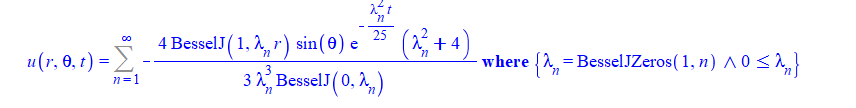I've used syntax (1) before many times and it works. Here is an example where all three syntax work

```pde:=diff(u(x,t),t)=k*diff(u(x,t),x\$2);
bc:=eval(diff(u(x,t),x),x=0)=0,u(L,t)=0;
ic:=u(x,0)=f(x);
sol:=pdsolve([pde,bc,ic],u(x,t));
```

pdsolve gives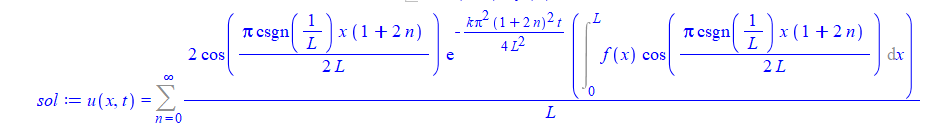Using syntax (2)

```pde:=diff(u(x,t),t)=k*diff(u(x,t),x\$2);
bc:=D(u)(0,t)=0,u(L,t)=0;
ic:=u(x,0)=f(x);
sol:=pdsolve([pde,bc,ic],u(x,t));```gives same answer as (1) and using syntax (3)

```pde:=diff(u(x,t),t)=k*diff(u(x,t),x\$2);
bc:=D*u(0,t)=0,u(L,t);
ic:=u(x,0)=f(x);
sol:=pdsolve([pde,bc,ic],u(x,t));
```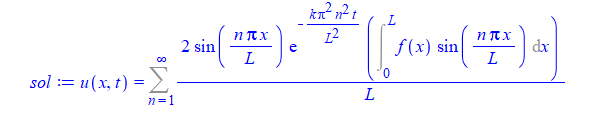The answer also looks like different and simpler, but I assume they are equivalent for now without looking too much into it.

Which syntax should one use as now I am really confused.

It looks like (3) is the one that should be used? Why the others did not work on first example? i.e. pdsolve did not give an answer at all?  And if (1,2,3) syntax are supposed to be equivalent, whysecond example gives slightly different looking answer when using one syntax vs. the other?

And finally to make things more confusing, here is an example where syntax (3) does not work, but syntax 1 and 2 work:

```#articolo example 8.4.3
restart;
pde := diff(u(x, t), t) = (1/20)*(diff(u(x, t), x, x))+t;
bc := u(0, t) = 5, (u(1, t)+ D(u)(1, t)) = 10;
ic:= u(x, 0) = -40*x^2*(1/3)+45*x*(1/2)+5;
pdsolve([pde, bc,ic], u(x, t))
```

```restart;
pde := diff(u(x, t), t) = (1/20)*(diff(u(x, t), x, x))+t;
bc := u(0, t) = 5, (u(1, t)+eval(diff(u(x,t),x),x=1))  = 10;
ic:= u(x, 0) = -40*x^2*(1/3)+45*x*(1/2)+5;
pdsolve([pde, bc,ic], u(x, t))
```

```restart;
pde := diff(u(x, t), t) = (1/20)*(diff(u(x, t), x, x))+t;
bc := u(0, t) = 5, (u(1, t)+ D*u(1, t)) = 10;
ic:= u(x, 0) = -40*x^2*(1/3)+45*x*(1/2)+5;
pdsolve([pde, bc,ic], u(x, t))
```

does not work.

Clearly there is something I do not understand between these 3 syntaxes and when to use which.

Using 265 version

```Physics:-Version()

10:41 hours, version in the MapleCloud: 265, version

installed in this computer: not installed```

## Can someone help me to write a code where beta is ...

In this code k=2 and Beta=2 has been cconsideredStep 1  Integrated supply chain

THE EXPECTED total PROFIT FUNCTION w r t LINEAR DEMAND(1)(2)

FIRST PUT ALL PARAMETER VALUES AND THEN GO FOR THE DIFFERENTIATION

RUN THE OPTIMALITY AND THE SUBROUTINES FOR DIFFERENT Beta and K values

CASE 1:   Put k = 2 and=2(3)(4)(5)(6)(7)

Calculate the Value of q(8)(9)

Step 2  Decentralized supply chain

Retailer's profit(10)(11)(12)Optimal q value for decentralized supply chain(13)

Manufacture's Profit(14)(15)

Total Wholesale Profit(16)(17)

step 3 find the t value(18)(19)

Hence range of t is 17.31 to 20.28

## how to collect denominator ...

I have
a:=-(diff(x(t), t))^2*h^2/(x(t)^2-2*d*x(t)+d^2+h^2)^(3/2);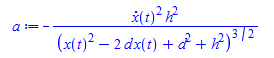How do you collect the denominator to get